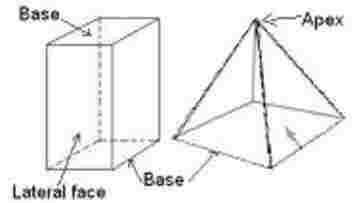# Quadrangular Prism Area, Lateral Area and Volume Calculation

Calculate the area, lateral area and volume of a quadrangle prism. The prism has quadrangles bases are called Quadrangular Prism.Lateral Area: I want to calculate : Select Lateral Area Height Perimeter Units Select Unit meters centimeters feet inches Perimeter of base : Height : Lateral Area :

 Total Area: I want to calculate : Select Total Area Lateral Area Area of base Units Select Unit meters centimeters feet inches Lateral Area : Area of base : Total area :

 Volume: I want to calculate : Select Volume height Area of base Units Select Unit meters centimeters feet inches Area of the base : Height : Volume :
We have following formulas in calculations:
Lateral Area           = Perimeter_of_base x Height
Perimeter of base = Lateral_Area / Height
Hight                     = Lateral_Area / Perimeter_of_base

Total Area              = Lateral_area + ( 2 x Area_of_the_base)
Lateral_area          = Total Area - ( 2 x Area_of_the_base)
Area of the base   = (Total Area - Lateral_area) / 2

Volume                 = Area_of_the base x Height
Height                  = Volume / Area_of_the base
Area_of_the base  = Volume / Height